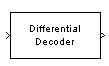Documentation

Differential Decoder

Decode binary signal using differential coding

Library

Source CodingDescription

The Differential Decoder block decodes the binary input signal. The output is the logical difference between the consecutive input element within a channel. More specifically, the block's input and output are related by

m(i0) = d(i0) XOR Initial condition parameter value

m(ik) = d(ik) XOR d(ik-1)

where

• d is the differentially encoded input.

• m is the output message.

• ik is the kth element.

• XOR is the logical exclusive-or operator.

This block accepts a scalar, column vector, or matrix input signal and treats columns as channels.

Parameters

Initial conditions

The logical exclusive-or of this value with the initial input value forms the initial output value.

Supported Data Type

PortSupported Data Types
In
• double

• single

• boolean

• integer

• fixed-point

Out
• double

• single

• boolean

• integer

• fixed-point

References

 Couch, Leon W., II, Digital and Analog Communication Systems, Sixth edition, Upper Saddle River, N. J., Prentice Hall, 2001.

Pair Block

Differential Encoder